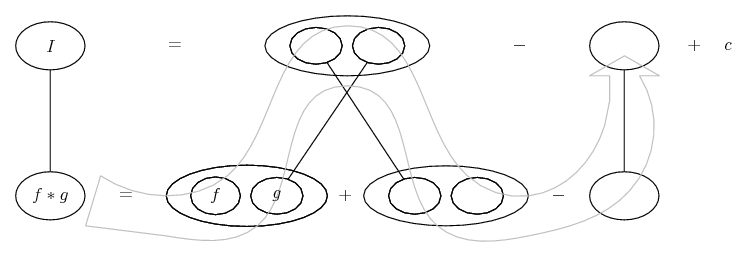# Thread: Integration by Parts

1.Originally Posted by mkeg1I am confused because the original problem gives the integral with n=0,1,2,.... then it asks for a recurrence formula for y sub k to y sub k-1 for k 1,2,3,.... am I still plugging in the same number?
You are meant to get an expression for $\displaystyle y_n$ in terms of $\displaystyle y_{n-1}$ and n. Please go back and carefully review the thread. Everything is there, all you have to do is put together.

2.Originally Posted by mr fantasticYou are meant to get an expression for $\displaystyle y_n$ in terms of $\displaystyle y_n$ and n. Please go back and carefully review the thread. Everything is there, all you have to do is put together.
$\displaystyle y_n$ should be in terms of $\displaystyle y_{n-1}$ and $\displaystyle n$3. $\displaystyle y_{n-1}=-t^ne^{-t} + n\int{e^{-t}t^{n-1}\,dt}$ for n=1,2,3....

?

4. Remember that $\displaystyle y_n = \int{e^{-t}t^{n}\,dt}$.

You have shown $\displaystyle \int{e^{-t}t^n\,dt} = -t^ne^{-t} + n\int{e^{-t}t^{n-1}\,dt}$.

So $\displaystyle y_n = -t^ne^{-t} + n\int{e^{-t}t^{n-1}\,dt}$.

Can you see that $\displaystyle \int{e^{-t}t^{n-1}\,dt}$ is almost the same as your original integral - the only difference being that $\displaystyle n$ is now $\displaystyle n-1$.

Surely this is $\displaystyle y_{n-1}$.

So what do you think $\displaystyle y_n$ is in terms of $\displaystyle y_{n-1}$?

5. $\displaystyle y_{n-1}=-t^{n-1}e^{-t} + (n-1)\int{e^{-t}t^{n-2}\,dt}$

6.Originally Posted by mkeg1$\displaystyle y_{n-1}=-t^ne^{-t} + n\int{e^{-t}t^{n-1}\,dt}$ for n=1,2,3....

?
Did you read post #12 at all? A lot of time is starting to be spent saying the same things. Please .... take some time to think about the probelm and what has been posted in this thread.

7. Yes, I read post 12, I am not very good at math as you can see. Maybe I just need to spend a little more time evaluating the problem

8.Originally Posted by mkeg1$\displaystyle y_{n-1}=-t^{n-1}e^{-t} + (n-1)\int{e^{-t}t^{n-2}\,dt}$
I suggest you read post number 19...

9.Originally Posted by Prove ItI suggest you read post number 19...
$\displaystyle y_n$ is a definite integral not an indefinite integral.

10. $\displaystyle y_n = -t^ne^{-t} + n\int{e^{-t}t^{n-1}\,dt}$

Kind of a shot in the dark because I sill dont understand, but would i replace the integral part with y sub n-1?

11.Originally Posted by mkeg1since $\displaystyle dv=e^{-t}dt$ i took the integral and got $\displaystyle -(1/e^{t}loge)$

I know integral of e^x is e^x but doesn tthe negative sign change it?
That "log" is the natural logarithm and log(e)= 1!

I suspect you looked up the integral of $\displaystyle a^x$. You should know that $\displaystyle \int e^x dx= e^x+ C$ without having to look it up. If you let u= -x, then du= -dx so that $\displaystyle \int e^{-x}dx= -\int e^u du= -e^u+ C= -e^{x}+ C$.

12. Just in case a picture helps...... where (key in spoiler) ...

Spoiler:... is the product rule. Straight continuous lines differentiate downwards (integrate up) with respect to x. And,... is lazy integration by parts, doing without u and v.

Now, of course, state $\displaystyle y_{k}$ in terms of $\displaystyle y_{k-1}$ and k.
_________________________________________

Don't integrate - balloontegrate!

Balloon Calculus; standard integrals, derivatives and methods

Balloon Calculus Drawing with LaTeX and Asymptote!

13. Hey guys I really appreciate all the help.

I understand the integration, I just dont understand what to do when applying $\displaystyle y_n$in terms of $\displaystyle y_{n-1}$

14. Can you at least see the pattern in

$\displaystyle y_{1} = y_{0} - e^{-1}$

$\displaystyle y_{2} = 2y_{1} - e^{-1}$

$\displaystyle y_{3} = 3y_{2} - e^{-1}$

and write it as

$\displaystyle y_{k} =$ ... ?

(or $\displaystyle y_{n} =$ ... ?)

I don't think any more is required than that. (And the starting value, for which, see above.)

15.Originally Posted by mkeg1$\displaystyle y_n = -t^ne^{-t} + n\int{e^{-t}t^{n-1}\,dt}$

Kind of a shot in the dark because I sill dont understand, but would i replace the integral part with y sub n-1?
No. For what seems like the one millionth time: $\displaystyle y_n$ is a DEFINITE integral!

$\displaystyle \displaystyle y_n = \left[-t^ne^{-t}\right]_{0}^{1} + n \int_0^1 {e^{-t}t^{n-1}\,dt}$.

Now the integral on the right hand side can be replaced with $\displaystyle y_{n-1}$. The first term on the right hand side needs to be evaluated in the usual way.

#### Search Tags

integration, parts